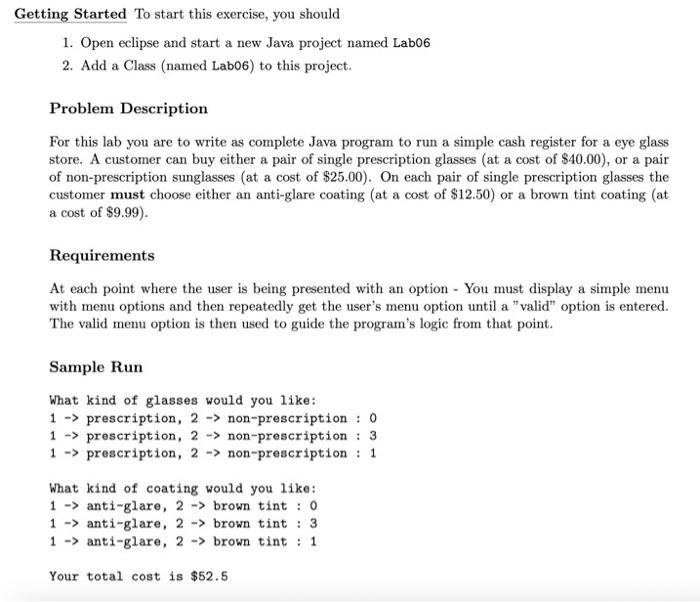# Question I use eclipse Getting Started To start this exercise, you should 1. Open eclipse and start a new Java project named Lab06 2. Add a Class (named Lab06) to this project. Problem Description For this lab you are to write as complete Java program to run a simple cash register for a eye glass store. A customer can buy either a pair of single prescription glasses (at a cost of $$\ 40.00$$ ), or a pair of non-prescription sunglasses (at a cost of $$\ 25.00$$ ). On each pair of single prescription glasses the customer must choose either an anti-glare coating (at a cost of $$\ 12.50$$ ) or a brown tint coating (at a cost of $$\ 9.99)$$. Requirements At each point where the user is being presented with an option - You must display a simple menu with menu options and then repeatedly get the user's menu option until a "valid" option is entered. The valid menu option is then used to guide the program's logic from that point. Sample Run What kind of glasses would you like: $$1 \rightarrow$$ prescription, $$2 \rightarrow$$ non-prescription : 0 $$1 \rightarrow$$ prescription, $$2 \rightarrow$$ non-prescription : 3 $$1 \rightarrow$$ prescription, 2 non-prescription : 1 What kind of coating would you like: $$1 \rightarrow$$ anti-glare, $$2 \rightarrow$$ brown tint : 0 $$1 \rightarrow$$ anti-glare, $$2 \rightarrow$$ brown tint : 3 $$1 \rightarrow$$ anti-glare, $$2 \rightarrow$$ brown tint : 1 Your total cost is $$\ 52.5$$ARNDWV The Asker · Computer Science
I use eclipseTranscribed Image Text: Getting Started To start this exercise, you should 1. Open eclipse and start a new Java project named Lab06 2. Add a Class (named Lab06) to this project. Problem Description For this lab you are to write as complete Java program to run a simple cash register for a eye glass store. A customer can buy either a pair of single prescription glasses (at a cost of $$\ 40.00$$ ), or a pair of non-prescription sunglasses (at a cost of $$\ 25.00$$ ). On each pair of single prescription glasses the customer must choose either an anti-glare coating (at a cost of $$\ 12.50$$ ) or a brown tint coating (at a cost of $$\ 9.99)$$. Requirements At each point where the user is being presented with an option - You must display a simple menu with menu options and then repeatedly get the user's menu option until a "valid" option is entered. The valid menu option is then used to guide the program's logic from that point. Sample Run What kind of glasses would you like: $$1 \rightarrow$$ prescription, $$2 \rightarrow$$ non-prescription : 0 $$1 \rightarrow$$ prescription, $$2 \rightarrow$$ non-prescription : 3 $$1 \rightarrow$$ prescription, 2 non-prescription : 1 What kind of coating would you like: $$1 \rightarrow$$ anti-glare, $$2 \rightarrow$$ brown tint : 0 $$1 \rightarrow$$ anti-glare, $$2 \rightarrow$$ brown tint : 3 $$1 \rightarrow$$ anti-glare, $$2 \rightarrow$$ brown tint : 1 Your total cost is $$\ 52.5$$
More
Transcribed Image Text: Getting Started To start this exercise, you should 1. Open eclipse and start a new Java project named Lab06 2. Add a Class (named Lab06) to this project. Problem Description For this lab you are to write as complete Java program to run a simple cash register for a eye glass store. A customer can buy either a pair of single prescription glasses (at a cost of $$\ 40.00$$ ), or a pair of non-prescription sunglasses (at a cost of $$\ 25.00$$ ). On each pair of single prescription glasses the customer must choose either an anti-glare coating (at a cost of $$\ 12.50$$ ) or a brown tint coating (at a cost of $$\ 9.99)$$. Requirements At each point where the user is being presented with an option - You must display a simple menu with menu options and then repeatedly get the user's menu option until a "valid" option is entered. The valid menu option is then used to guide the program's logic from that point. Sample Run What kind of glasses would you like: $$1 \rightarrow$$ prescription, $$2 \rightarrow$$ non-prescription : 0 $$1 \rightarrow$$ prescription, $$2 \rightarrow$$ non-prescription : 3 $$1 \rightarrow$$ prescription, 2 non-prescription : 1 What kind of coating would you like: $$1 \rightarrow$$ anti-glare, $$2 \rightarrow$$ brown tint : 0 $$1 \rightarrow$$ anti-glare, $$2 \rightarrow$$ brown tint : 3 $$1 \rightarrow$$ anti-glare, $$2 \rightarrow$$ brown tint : 1 Your total cost is $$\ 52.5$$